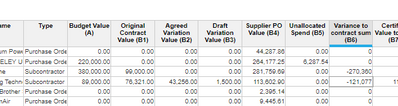# Formula HelpHi - Please could you help me tidy this forumla so that its accepted by Anaplan?

IF Type = Supplier Type.Purchase Order (IF 'Forecasted total Value £ (E)' - 'Supplier PO Value (B4)' > 0 THEN 0 ELSE 'Forecasted total Value £ (E)' - 'Supplier PO Value (B4)')

OR Type = Supplier Type.Subcontractor (IF 'Forecasted total Value £ (E)' - 'Original Contract Value (B1)' -  'Agreed Variation Value (B2)' - 'Draft Variation Value (B3)' THEN 0 ELSE 'Forecasted total Value £ (E)' - 'Original Contract Value (B1)' - 'Agreed Variation Value (B2)' - 'Draft Variation Value (B3)')Tagged:

•Try the below formula:

if Type = Supplier Type.Purchase Order then IF ('Forecasted total Value £ (E)' - 'Supplier PO Value (B4)') > 0 THEN 0 ELSE 'Forecasted total Value £ (E)' - 'Supplier PO Value (B4)' else if Type = Supplier Type.Subcontractor then IF ('Forecasted total Value £ (E)' - 'Original Contract Value (B1)' -  'Agreed Variation Value (B2)' - 'Draft Variation Value (B3)')>0 THEN 0 ELSE 'Forecasted total Value £ (E)' - 'Original Contract Value (B1)' - 'Agreed Variation Value (B2)' - 'Draft Variation Value (B3)' else 0

Thanks

•Pls try using below code.

IF Type = Supplier Type.Purchase Order THEN (IF 'Forecasted total Value £ (E)' - 'Supplier PO Value (B4)' > 0 THEN 0 ELSE 'Forecasted total Value £ (E)' - 'Supplier PO Value (B4)') ELSE 0 OR IF Type = Supplier Type.Subcontractor THEN (IF 'Forecasted total Value £ (E)' - 'Original Contract Value (B1)' -  'Agreed Variation Value (B2)' - 'Draft Variation Value (B3)' THEN 0 ELSE 'Forecasted total Value £ (E)' - 'Original Contract Value (B1)' - 'Agreed Variation Value (B2)' - 'Draft Variation Value (B3)') ELSE 0

Thanks,

Rahul

•Thank you!!

•In your formula below, OR condition used between two numbers as the LHS and RHS returns the number.

Also, Second if condition is returning number but If will expect Boolean value. Please verify.

IF Type = Supplier Type.Purchase Order THEN (IF 'Forecasted total Value £ (E)' - 'Supplier PO Value (B4)' > 0 THEN 0 ELSE 'Forecasted total Value £ (E)' - 'Supplier PO Value (B4)') ELSE 0 OR IF Type = Supplier Type.Subcontractor THEN (IF 'Forecasted total Value £ (E)' - 'Original Contract Value (B1)' -  'Agreed Variation Value (B2)' - 'Draft Variation Value (B3)' THEN 0 ELSE 'Forecasted total Value £ (E)' - 'Original Contract Value (B1)' - 'Agreed Variation Value (B2)' - 'Draft Variation Value (B3)') ELSE 0